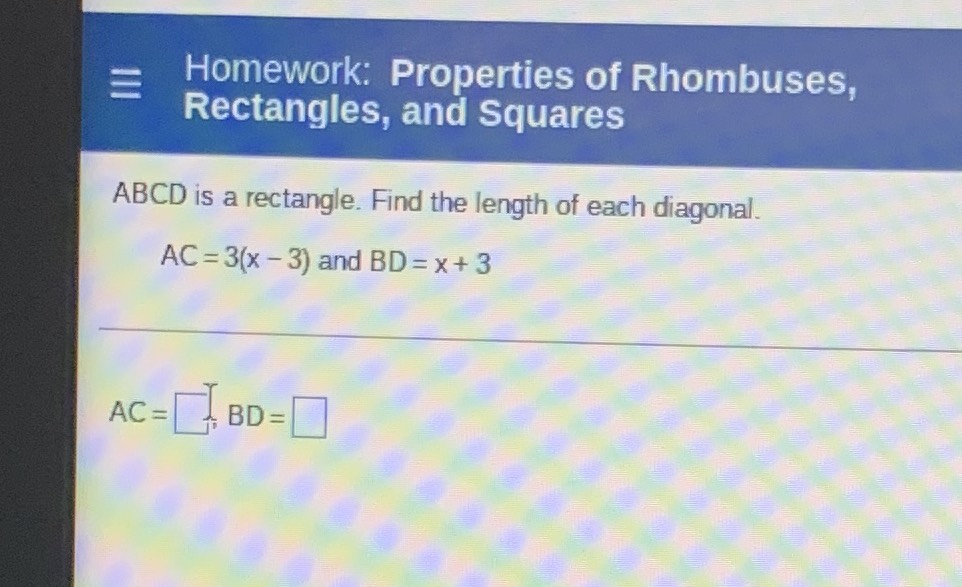### ¿Todavía tienes preguntas de matemáticas?

Pregunte a nuestros tutores expertos
Algebra
Pregunta$$A B C D$$ is a rectangle. Find the length of each diagonal.

$$A C = 3 ( x - 3 )$$ and $$B D = x + 3$$

$$AC = \square , BD = \square$$